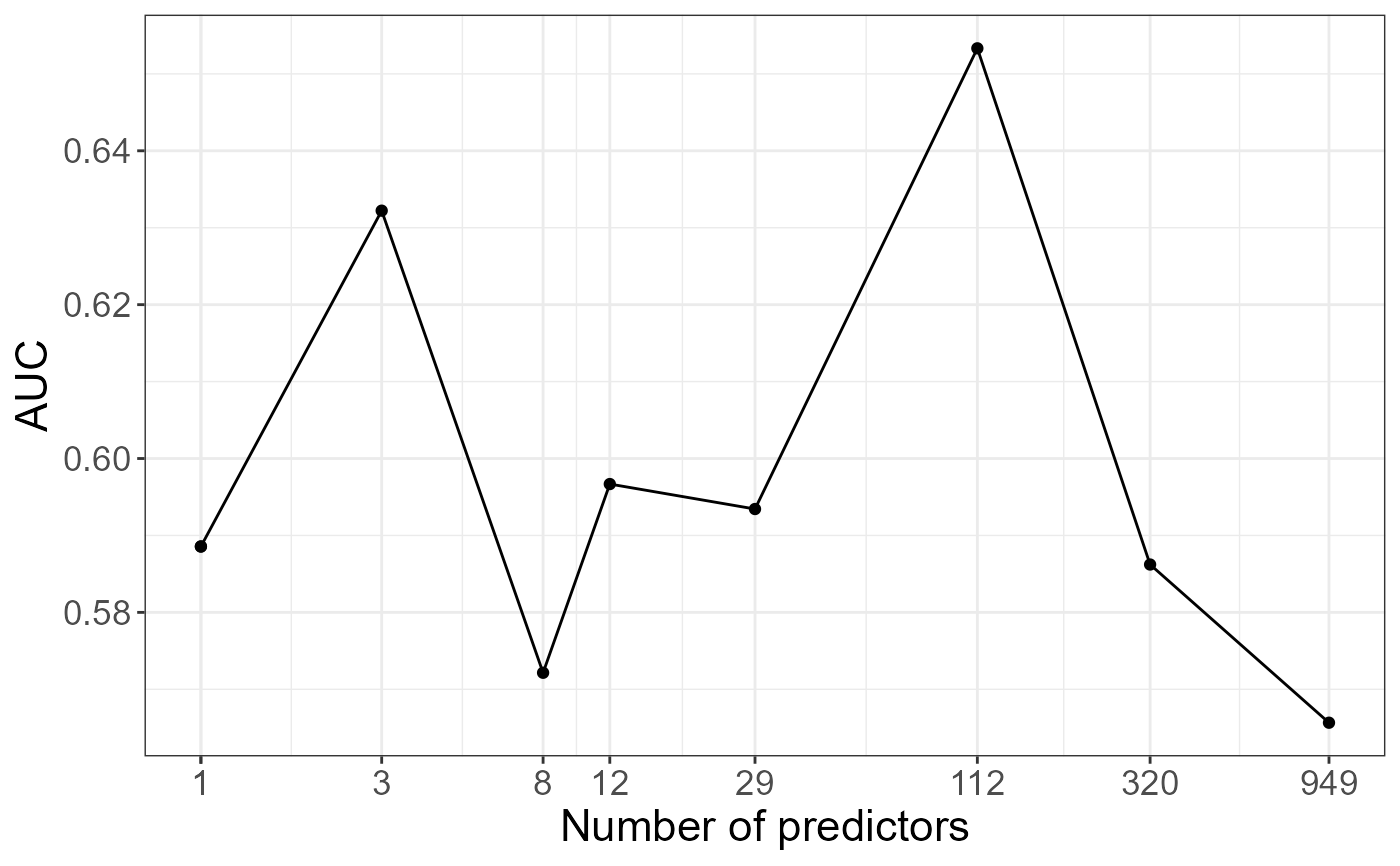Polygenic Risk Scores with possible clumping and thresholding.

snp_PRS(
G,
betas.keep,
ind.test = rows_along(G),
ind.keep = cols_along(G),
same.keep = rep(TRUE, length(ind.keep)),
lpS.keep = NULL,
thr.list = 0
)

G A FBM.code256 (typically $genotypes). You shouldn't have missing values. Also, remember to do quality control, e.g. some algorithms in this package won't work if you use SNPs with 0 MAF. Numeric vector of weights associated with each SNP corresponding to ind.keep. You may want to see big_univLinReg or big_univLogReg. The individuals on whom to project the scores. Default uses all. Column (SNP) indices to use (if using clumping, the output of snp_clumping). Default doesn't clump. A logical vector associated with betas.keep whether the reference allele is the same for G. Default is all TRUE (for example when you train the betas on the same dataset). Otherwise, use same_ref. Numeric vector of -log10(p-value) associated with betas.keep. Default doesn't use thresholding. Threshold vector on lpS.keep at which SNPs are excluded if they are not significant enough. Default doesn't use thresholding. Value A matrix of scores, where rows correspond to ind.test and columns correspond to thr.list. Examples test <- snp_attachExtdata() G <- big_copy(test$genotypes, ind.col = 1:1000) CHR <- test$map$chromosome[1:1000] POS <- test$map$physical.position[1:1000] y01 <- test$fam$affection - 1 # PCA -> covariables obj.svd <- snp_autoSVD(G, infos.chr = CHR, infos.pos = POS)
#> #> Phase of clumping (on MAF) at r^2 > 0.2.. keep 952 SNPs. #> Discarding 0 variant with MAC < 10. #> #> Iteration 1: #> Computing SVD.. #> 26 outlier variants detected.. #> #> Iteration 2: #> Computing SVD.. #> 0 outlier variant detected.. #> #> Converged!
# train and test set ind.train <- sort(sample(nrow(G), 400)) ind.test <- setdiff(rows_along(G), ind.train) # 117 # GWAS gwas.train <- big_univLogReg(G, y01.train = y01[ind.train], ind.train = ind.train, covar.train = obj.svd$u[ind.train, ]) # clumping ind.keep <- snp_clumping(G, infos.chr = CHR, ind.row = ind.train, S = abs(gwas.train$score)) # -log10(p-values) and thresolding summary(lpS.keep <- -predict(gwas.train)[ind.keep])
#> Min. 1st Qu. Median Mean 3rd Qu. Max. #> 0.000058 0.127537 0.339442 0.470071 0.663226 6.312297
thrs <- seq(0, 4, by = 0.5) nb.pred <- sapply(thrs, function(thr) sum(lpS.keep > thr)) # PRS prs <- snp_PRS(G, betas.keep = gwas.train\$estim[ind.keep], ind.test = ind.test, ind.keep = ind.keep, lpS.keep = lpS.keep, thr.list = thrs) # AUC as a function of the number of predictors aucs <- apply(prs, 2, AUC, target = y01[ind.test]) library(ggplot2)
#> Warning: package 'ggplot2' was built under R version 3.6.3
qplot(nb.pred, aucs) + geom_line() + scale_x_log10(breaks = nb.pred) + labs(x = "Number of predictors", y = "AUC") + theme_bigstatsr()